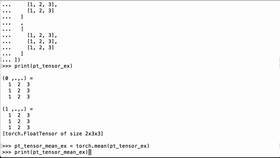This Lesson is for subscribersUnlock this lesson NOW!
• Get expert advice on how to Calculate The Mean Value Of All Elements In A PyTorch Tensor
• Learn Deep Learning Technology Like Your Career Depends On It!

## Calculate The Mean Value Of All Elements In A PyTorch Tensor

Calculate the Mean value of all elements in a tensor by using the PyTorch mean operation

Type: PRO     Duration: 2:01   Technologies: PyTorch, Python

Page Sections: Video  |  Code  |  Transcript

### < > Code:

You must be a Member to view code

Access all courses and lessons, gain confidence and expertise, and learn how things work and how to use them.

### Transcript:

This Transcript is for subscribersUnlock this lesson's Transcript NOW!
• Get expert advice on how to Calculate The Mean Value Of All Elements In A PyTorch Tensor